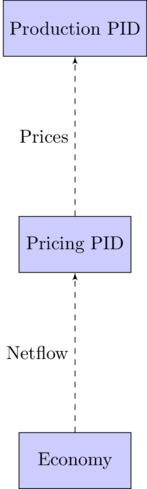04/09/2015

## What am I doing

• Less than fully rational agents are hard

• Start from the opposite direction

• Use simple trial and error

## Who cares?

• Modeling Contribution

• "Suppose first that the market price is above the equilibrium price […] There is a surplus of the good: suppliers are unable to sell all they want at the going price. […] They respond to the surplus by cutting their prices. Prices continue to fall until the market reaches the equilibrium."

• Robustness Check

## The Thermostat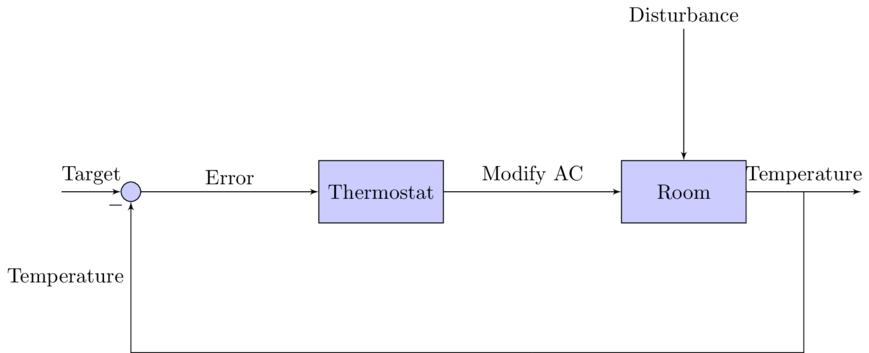## Feedback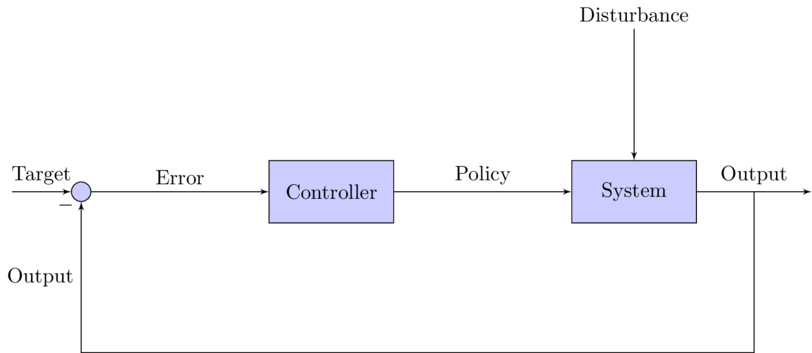## The agent diagram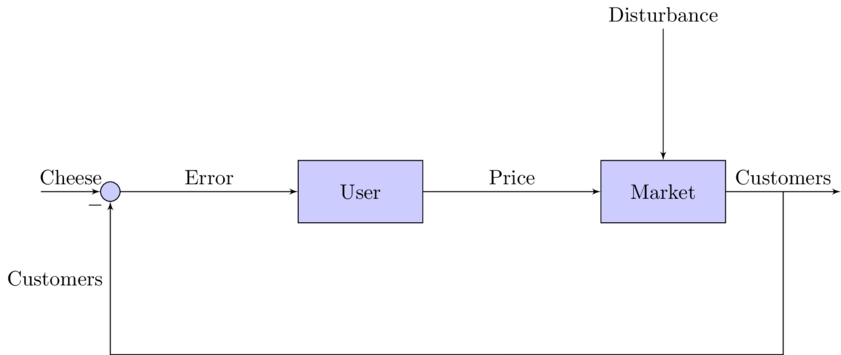## Feedback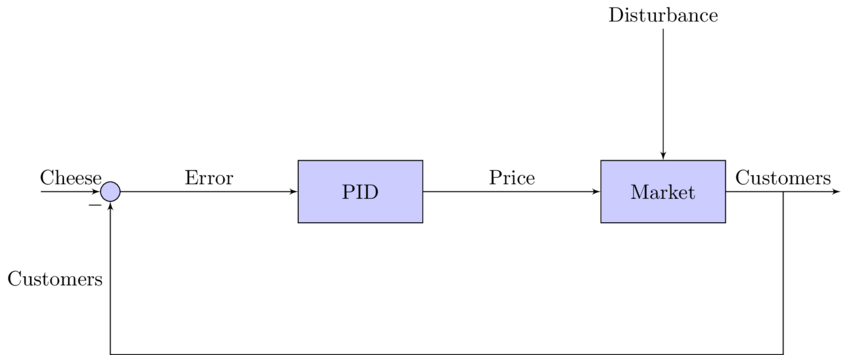## Formally

• A seller wants to sell $$y^*$$ kilos of cheese each day. Today he charged price $$p_t$$ and sold $$y_t$$ kilos. How should he sets tomorrow's price $$p_{t+1}$$

• $$e_t = y^* -y_t$$

• $$p_{t+1} = a e_t + b \sum_0^t e_{\tau} d\tau + c \frac{de_t}{d_t} + p_0$$

## Why PI(D) Controllers

• Absence of model knowledge

• Assume unsophisticated users

• Simple to code

• Simple to fit

## Cyber-ECB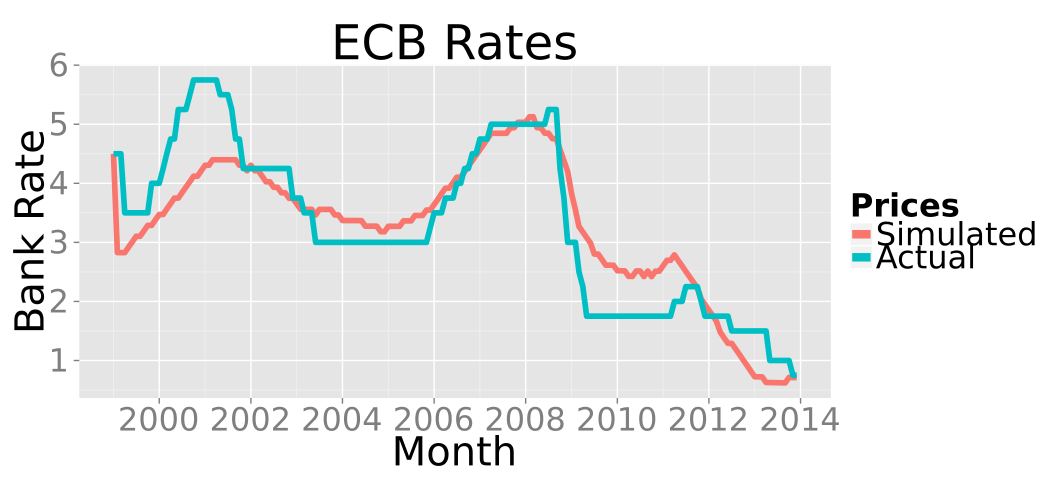## Alternatives

• Reactive/Subsumption Architecture

• Zero-Intelligence (Plus)

• Probe and Adjust

• Gintis

## Adapting over Learning - Change in Endowment

• Making 50 kilos of cheese a day require action over multiple markets: milk, workers, sales. How do we generalize PID pricing over multiple markets?

• How do we decide how many kilos of cheese to produce in order to maximize profits?

## Marginal Maximizer

• Profits are maximized when marginal benefits equal marginal costs

• $$\text{Marginal Benefit: } p_t = w_t \text{ :Marginal Costs}$$

## We know how to deal with sliders

• Use a PID controller to find how for which production target $$p_t = w_t$$

• Lower Frequency

## Decisions are fed in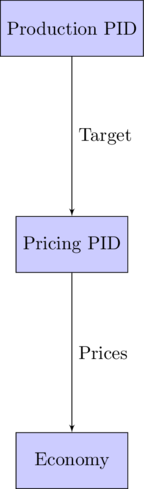## Information feeds back